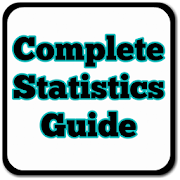# Complete Statistics Guide (OFFLINE) - Lumos Educational App Store3.91
Price -fREE
\$0

#### DESCRIPTION:

This Statistics guide will cover the important concepts of Statistics syllabus. It contains chapters discussing all the basic concepts of Statistics with suitable examples. We Have Complied Complete Statistics Syllabi including Chapters like: Adjusted R-Squared Analysis of Variance Arithmetic Mean Arithmetic Median Arithmetic Mode Arithmetic Range Bar Graph Best Point Estimation Beta Distribution Binomial Distribution Black-Scholes model Boxplots Central limit theorem Chebyshev's Theorem Chi-squared Distribution Chi Squared table Circular Permutation Cluster sampling Co

#### OVERVIEW:

Complete Statistics Guide (OFFLINE) is a free educational mobile app By .It helps students in grades HS practice the following standards HSS.ID.A.2.

This page not only allows students and teachers download Complete Statistics Guide (OFFLINE) but also find engaging Sample Questions, Videos, Pins, Worksheets, Books related to the following topics.

1. HSS.ID.A.2 : Use statistics appropriate to the shape of the data distribution to compare center (median, mean) and spread (interquartile range, standard deviation) of two or more different data sets..

HS

HSS.ID.A.2

#### ADDITIONAL INFORMATION:

Developer:

Software Version: 1.0.2

Category:

### RELATED APPSEdSearch WebSearch